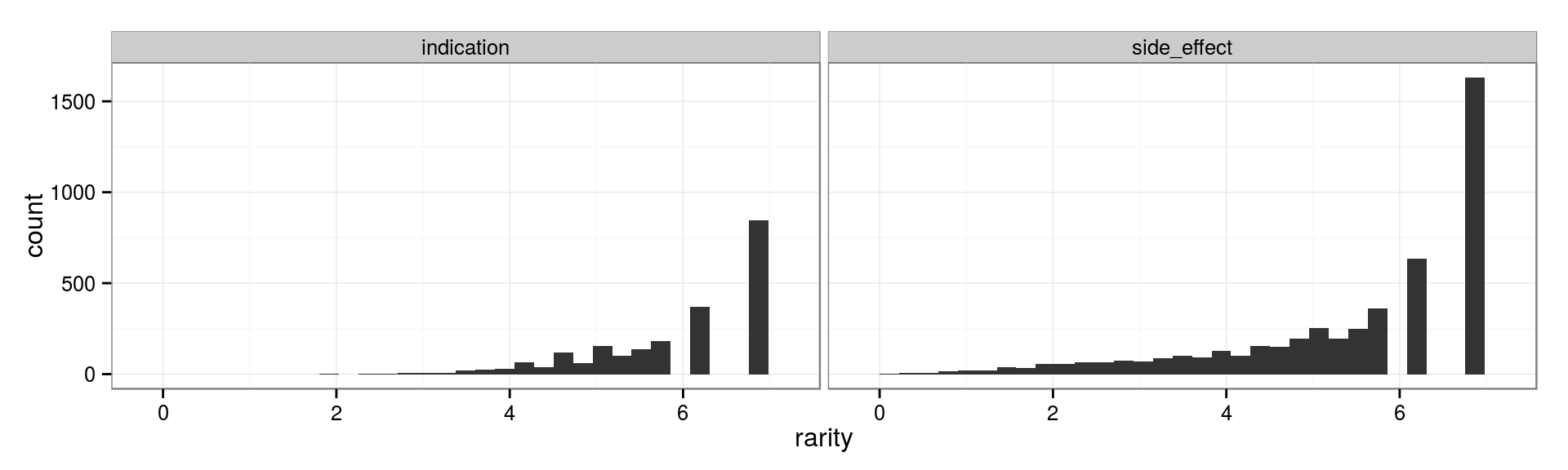Here, we compute the pairwise similarities between compounds. We compute drug-drug similarities separately based on three different types of information:

• side effects
• indications
• substructure

The method for calculating side effect and indication similarity is based on the method from a previous analysis (1). Substructure similarity is calculated externally using PubChem.

We use dplyr and reshape2 for data manipulation; ggplot2 and ggdendro for plotting; and DT for displaying html tables using the Javascript DataTables library.

``````library(reshape2)
library(dplyr)
library(ggplot2)
library(ggdendro)
library(DT)

options(stringsAsFactors=FALSE)
doMC::registerDoMC(cores = 6)

write.delim <- function(x, file, sep='\t', quote = FALSE, row.names=FALSE, na = '', ...) {
write.table(x = x, file = file, sep=sep, quote=quote, row.names=row.names, na=na, ...)
}``````

### Substructure Similarity

We use PubChem’s score matrix service. We use the 2D substructure search:

2D Similarity: Substructure key-based 2D Tanimoto similarity. Scores are in the range [0 .. 100].

PubChem provides additional explanation of the keys used to describe substructure:

There are 881 substructure-keys (skeys) in each fingerprint. Each bit in the fingerprint represents the presence (or absence) of a particular chemical substructure (e.g., a carboxylic acid) or a particular count of the same. These skeys are similar in nature to the well-known MDL MACCS skeys fingerprints.

To perform the search, we selected Upload a file with IDs… under `ID List` and choose `sider_compounds_pubchem.txt`. We selected `Id-Id Score` for output format and `gzip`for compression.

``````structure.df <- file.path('..', 'data', 'pubchem_score_matrix_2d.tab.gz') %>%
read.table(col.names = c('compound_1', 'compound_2', 'substructure')) %>%
dplyr::mutate(substructure = substructure / 100) %>%
dplyr::filter(compound_1 != compound_2)``````

### Read Processed SIDER 2 Data

Previousely, we processed data from the from the SIDER 2 resource (2). Here, we read the data and convert from a pairwise format to a binary matrix.

``````sider.df <- file.path('..', 'data', 'sider2-processed.txt') %>% read.delim(na.strings='')

# Convert into matrixes
se.mat <- xtabs(formula = ~ pubchem_cid + concept_id ,
data = dplyr::filter(sider.df, type == 'side_effect')) %>% as.matrix()
ind.mat <- xtabs(formula = ~ pubchem_cid + concept_id ,
data = dplyr::filter(sider.df, type == 'indication')) %>% as.matrix()``````

## Compute Side Effect and Indication Similarity

We adopted a similarity metric that assesses the extent of concept (either side effect or indication) overlap between two compounds. The method weights concepts based on their rarity (relating to few compounds) and underrepresentation (dissimilarity compared to other concetps).

### Rarity Weighting

Rarity weighting was performed as previousely described (1):

Our weighting scheme consists of two parts, a rareness weight and a correlation weight. The recorded side effects vary greatly in abundance: some, like megaloblastic 4 anaemia, occur in only a few package inserts, while others, like dizziness, appear in most. To be able to account for this, we examined the relation between side-effect frequency and the probability of sharing a drug target within a reference set of 502 drugs with 4857 known human drug–target relations (Fig. S1). We observed an inverse correlation between side-effect frequency and the likelihood of two drugs sharing a protein target. Consequently, the rareness weight for a side effect, \(r_i\), is defined as the negative logarithm of the side-effect frequency (Fig. S1D). (This frequency refers to the fraction of package inserts that feature a certain side effect, not the relative occurrence of the side effect in patients.)

``````rarity.se.vec <- -log(colMeans(se.mat))
rarity.ind.vec <- -log(colMeans(ind.mat))

dplyr::bind_rows(
data.frame(rarity=rarity.se.vec, type='side_effect'),
data.frame(rarity=rarity.ind.vec, type='indication')) %>%
ggplot(aes(x = rarity)) + theme_bw() +
facet_grid(. ~ type) +
geom_histogram()``````### Underrepresentation Weighting

Underrepresentation weighting was also performed as previousely described (1), except that Ward’s method (3) was used for heirarchical clustering:

Not all side effects are independent of each other; for example, 90% of drugs that cause nausea also cause vomiting. We correct for this redundancy by weighting side effects in a manner analogous to the down-weighting of similar protein sequences within multiple alignments (4) (Fig. S1C). In order to determine the correlation weight, the correlation of side effects was determined by clustering all side effects according to their assigned drugs using a Tanimoto/Jacquard score to compute a distance matrix: The distance between two drugs [side effects] was calculated by dividing the number of drugs that feature both side effects by the number of drugs that have either side effect associated. The Gerstein–Sonnhammer–Chothia algorithm (4) was used to compute weights based on a hierarch[ic]al clustering with the aforementioned distance matrix (5).

``````# Uniform wieghting until a better scheme is implemented
underrep.se.vec <- rep(1, ncol(se.mat))
underrep.ind.vec <- rep(1, ncol(ind.mat))

GitHubScript <- function(...) {
# Source a script from GitHub
library(RCurl)
github.url <- file.path('https://raw.githubusercontent.com', ...)
script <- RCurl::getURL(github.url)
eval(parse(text = script), envir = .GlobalEnv)
}

CalcUnderrep <- function(mat) {
# Returns underrepresentation weight for each column
col.dist <- stats::dist(t(mat), method = 'binary')
col.clust <- hclust(col.dist, method = 'ward.D2')
col.dendro <- as.dendrogram(col.clust)
GitHubScript('antoine-lizee', 'R-GSC', 'master', 'GSC.R')
gsc.weight <- GSC(col.dendro)
list(dist = col.dist, clust = col.clust, dendro = col.dendro, weight = gsc.weight)
}

underrep.se <- CalcUnderrep(se.mat)
underrep.ind <- CalcUnderrep(ind.mat)

ggdendro.se <- ggdendro::dendro_data(underrep.se\$clust)
ggdendro.ind <- ggdendro::dendro_data(underrep.ind\$clust)

dendro.df <- dplyr::bind_rows(
ggdendro.se %>% ggdendro::segment() %>% dplyr::mutate(type='Side effects'),
ggdendro.ind %>% ggdendro::segment() %>% dplyr::mutate(type='Indications'))

leaf.df <- dplyr::bind_rows(
ggdendro.se %>% ggdendro::label() %>% dplyr::left_join(
y = data.frame(label = names(underrep.se\$weight), gsc_weight = underrep.se\$weight)) %>%
dplyr::mutate(type='Side effects'),
ggdendro.ind %>% ggdendro::label() %>% dplyr::left_join(
y = data.frame(label = names(underrep.ind\$weight), gsc_weight = underrep.ind\$weight)) %>%
dplyr::mutate(type='Indications'))

# plot denogrograms
dendro.df %>% ggplot(aes(x=x, y=y)) + theme_bw() +
facet_grid( ~ type, scales = 'free_x', space = 'free_x') +
geom_segment(aes(xend=xend, yend=yend), alpha=0.25) +
geom_point(data = leaf.df, aes(color=gsc_weight), alpha=0.04, size=3) +
xlab(NULL) + ylab('Distance') +